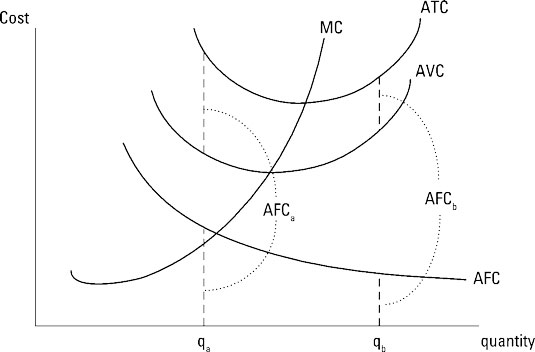##### Managerial Economics For Dummies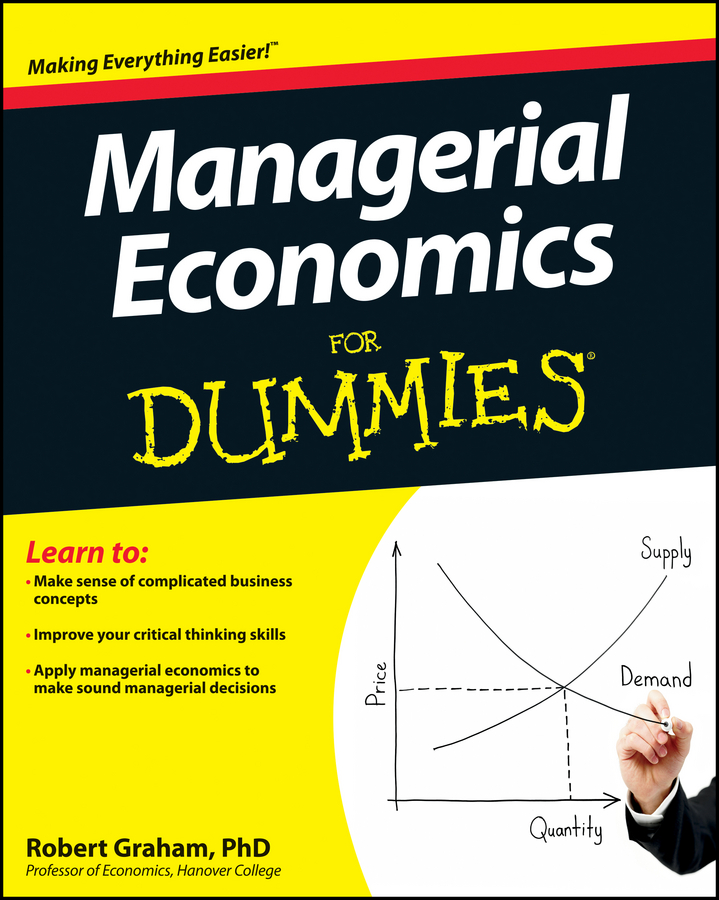As a manager you frequently want to know the cost per unit associated with producing a good, because you can use this information to establish your product’s price and determine your profit per unit. If you want to know cost per unit, average cost is what you need to know.

## Average total cost

Average total cost (ATC) is the total cost per unit of output. It’s determined by dividing the total cost equation by the quantity of output. Using the total cost equation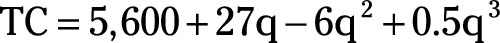average total cost equals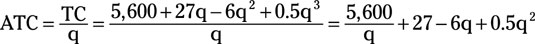where q is the quantity of output produced.

Marginal cost always intersects the minimum point on the average total cost curve. Thus, average total cost initially decreases, and then begins to increase, resulting in a U-shaped curve.

Average total cost has two components — average fixed cost and average variable cost. As an equation,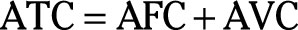## Average fixed cost

Average fixed cost (AFC) is fixed cost per unit of output and is determined by dividing total fixed cost by the quantity of output. In the total cost equationtotal fixed cost is \$5,600, so averaged fixed cost is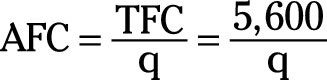Because the numerator of average fixed cost is a constant, while the denominator is the quantity of output produced, average fixed cost always decreases as the quantity of output produced increases.

## Average variable cost

Average variable cost (AVC) represents variable cost per unit of output and equals total variable cost divided by the quantity of output. Given total variable cost equals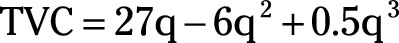average variable cost equals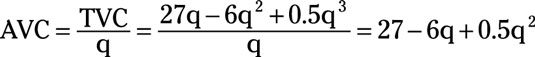Typically, average variable cost initially decreases, and then begins to increase, resulting in a U-shaped curve. Marginal cost intersects the minimum point on the average variable cost curve.

The illustration shows the average total cost, average fixed cost, average variable cost, and marginal cost curves. Note that the average fixed cost curve is always decreasing and also note that the difference between average total cost and average variable cost is average fixed cost — so AFCb at qb is less than AFCa at qa.

As you produce more output, average variable cost and average total cost get closer to one another. Finally, marginal cost intersects the minimum points on the average variable and average total cost curves.